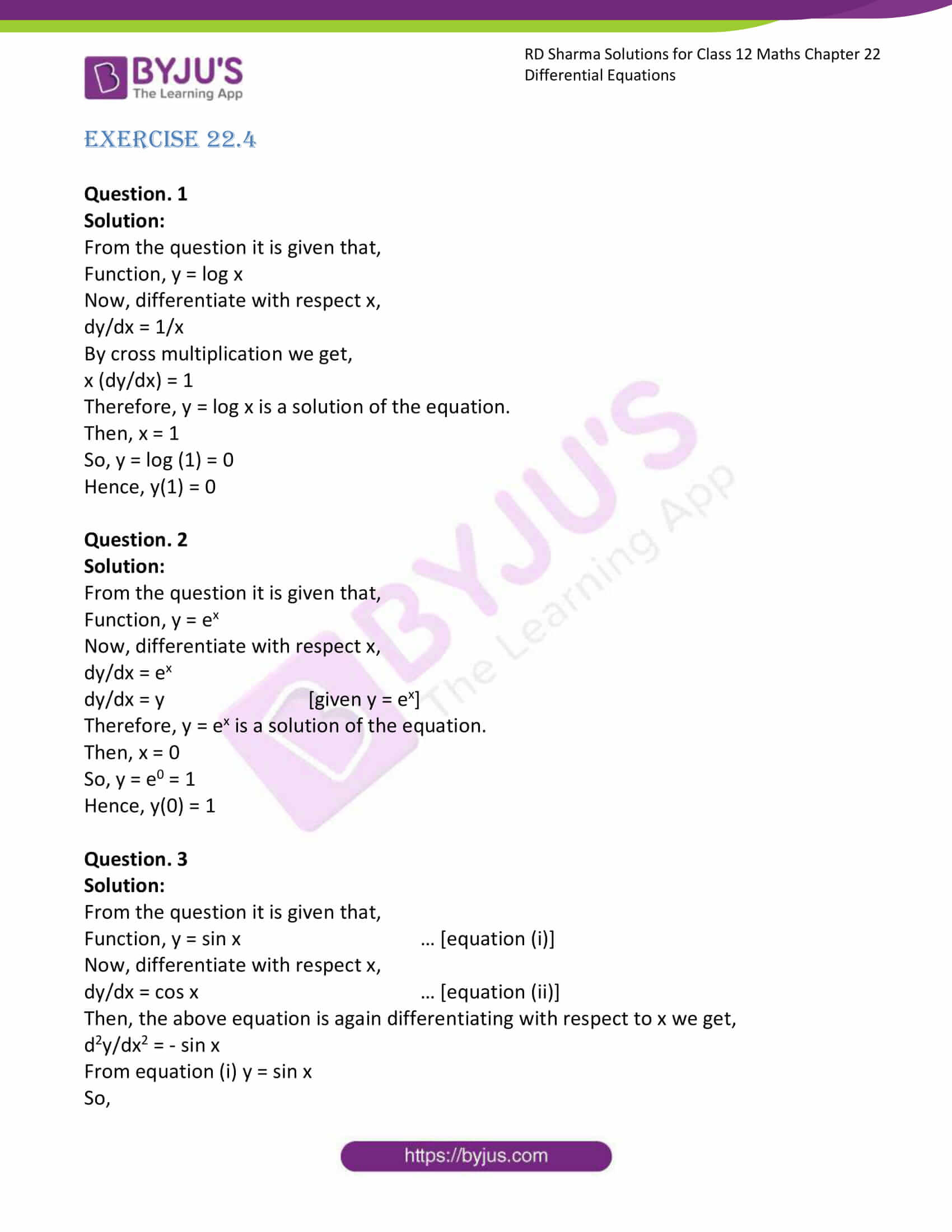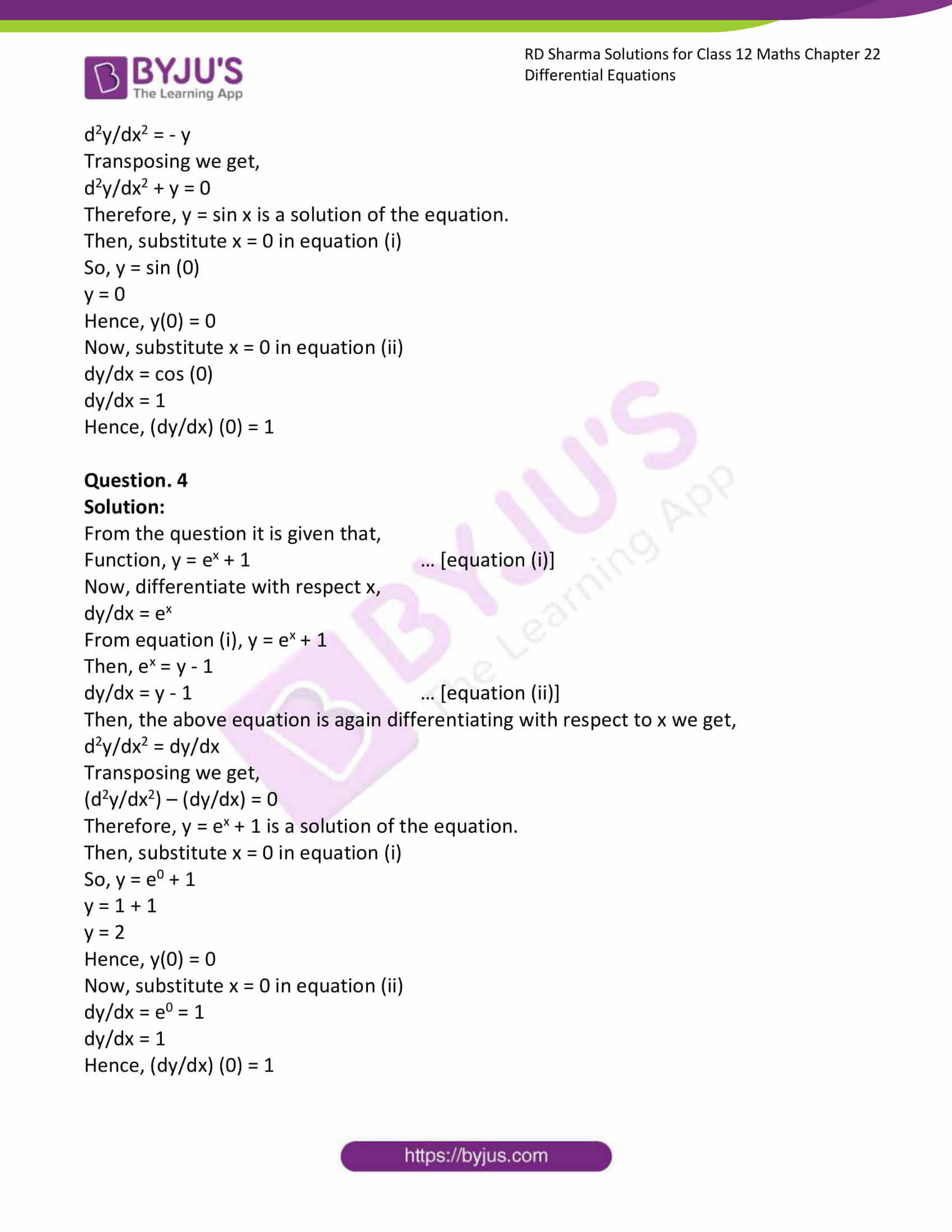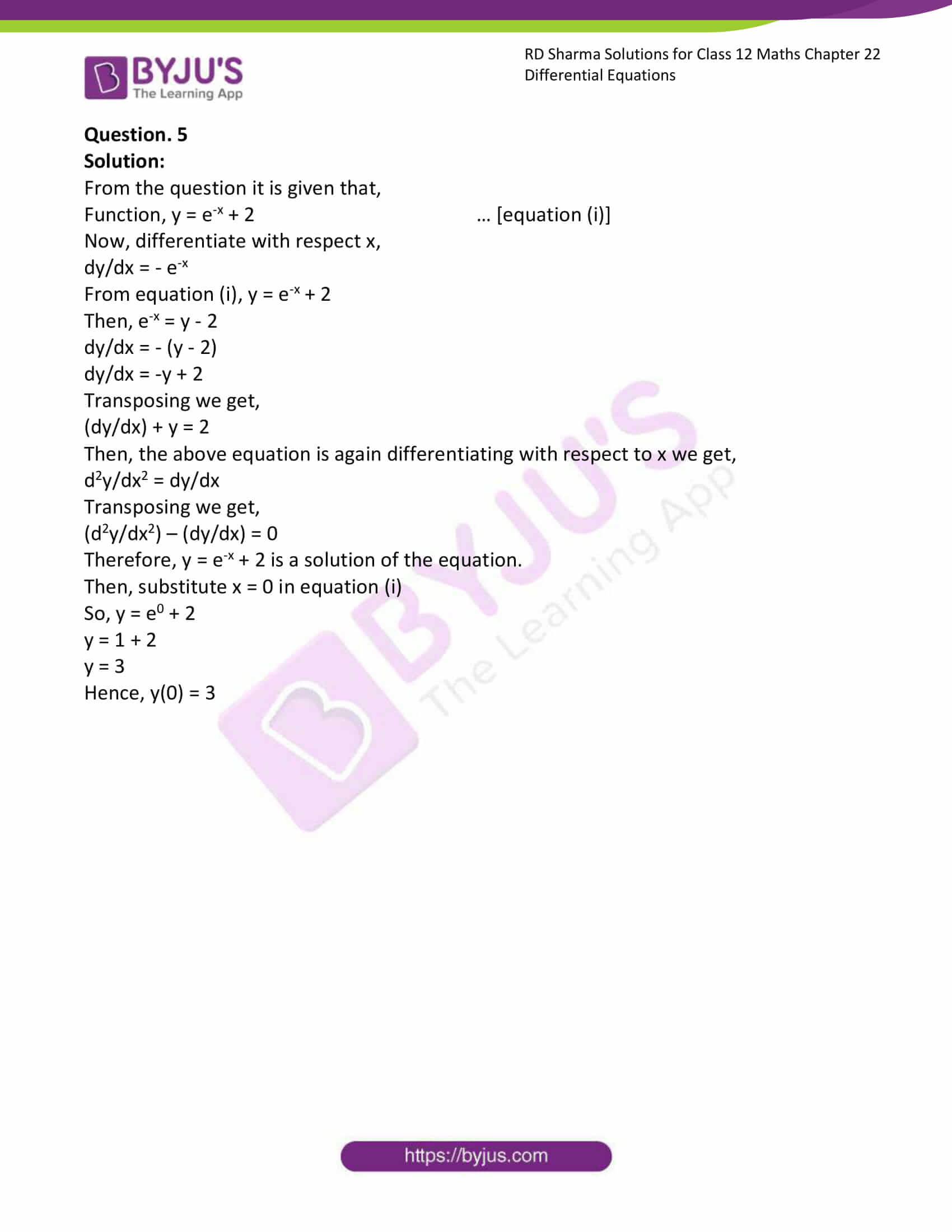# RD Sharma Solutions Class 12 Differential Equations Exercise 22.4

RD Sharma Solutions for Class 12 Maths Exercise 22.4 Chapter 22 Differential Equations are available here. When students feel stressed about searching for the most comprehensive and detailed RD Sharma Solutions for Class 12 Maths Chapter 22, we at BYJU’S, have prepared step-by-step solutions with detailed explanations. We advise students who want to score good marks in Maths, to go through these solutions and strengthen their knowledge.

## Download PDF of RD Sharma Solutions for Class 12 Maths Chapter 22 Exercise 4### Access RD Sharma Solutions for Class 12 Maths Chapter 22 Exercise 4

EXERCISE 22.4

Question. 1

Solution:

From the question it is given that,

Function, y = log x

Now, differentiate with respect x,

dy/dx = 1/x

By cross multiplication we get,

x (dy/dx) = 1

Therefore, y = log x is a solution of the equation.

Then, x = 1

So, y = log (1) = 0

Hence, y(1) = 0

Question. 2

Solution:

From the question it is given that,

Function, y = ex

Now, differentiate with respect x,

dy/dx = ex

dy/dx = y [given y = ex]

Therefore, y = ex is a solution of the equation.

Then, x = 0

So, y = e0 = 1

Hence, y(0) = 1

Question. 3

Solution:

From the question it is given that,

Function, y = sin x … [equation (i)]

Now, differentiate with respect x,

dy/dx = cos x … [equation (ii)]

Then, the above equation is again differentiating with respect to x we get,

d2y/dx2 = – sin x

From equation (i) y = sin x

So,

d2y/dx2 = – y

Transposing we get,

d2y/dx2 + y = 0

Therefore, y = sin x is a solution of the equation.

Then, substitute x = 0 in equation (i)

So, y = sin (0)

y = 0

Hence, y(0) = 0

Now, substitute x = 0 in equation (ii)

dy/dx = cos (0)

dy/dx = 1

Hence, (dy/dx) (0) = 1

Question. 4

Solution:

From the question it is given that,

Function, y = ex + 1 … [equation (i)]

Now, differentiate with respect x,

dy/dx = ex

From equation (i), y = ex + 1

Then, ex = y – 1

dy/dx = y – 1 … [equation (ii)]

Then, the above equation is again differentiating with respect to x we get,

d2y/dx2 = dy/dx

Transposing we get,

(d2y/dx2) – (dy/dx) = 0

Therefore, y = ex + 1 is a solution of the equation.

Then, substitute x = 0 in equation (i)

So, y = e0 + 1

y = 1 + 1

y = 2

Hence, y(0) = 0

Now, substitute x = 0 in equation (ii)

dy/dx = e0 = 1

dy/dx = 1

Hence, (dy/dx) (0) = 1

Question. 5

Solution:

From the question it is given that,

Function, y = e-x + 2 … [equation (i)]

Now, differentiate with respect x,

dy/dx = – e-x

From equation (i), y = e-x + 2

Then, e-x = y – 2

dy/dx = – (y – 2)

dy/dx = -y + 2

Transposing we get,

(dy/dx) + y = 2

Then, the above equation is again differentiating with respect to x we get,

d2y/dx2 = dy/dx

Transposing we get,

(d2y/dx2) – (dy/dx) = 0

Therefore, y = e-x + 2 is a solution of the equation.

Then, substitute x = 0 in equation (i)

So, y = e0 + 2

y = 1 + 2

y = 3

Hence, y(0) = 3